## ​41% of U.S. adults have very little confidence in newspapers. You randomly select 10 U.S. adults. Find the probability that the number of U

Question

​41% of U.S. adults have very little confidence in newspapers. You randomly select 10 U.S. adults. Find the probability that the number of U.S. adults who have very little confidence in newspapers is ​ (a) exactly​ five, (b) at least​ six, and​ (c) less than four.

in progress 0
4 months 2021-09-05T13:53:05+00:00 1 Answers 121 views 0

## Answers ( )

a) 0.2087 = 20.82% probability that the number of U.S. adults who have very little confidence in newspapers is exactly​ five.

b) 0.1834 = 18.34% probability that the number of U.S. adults who have very little confidence in newspapers is at least​ six.

c) 0.3575 = 35.75% probability that the number of U.S. adults who have very little confidence in newspapers is less than four.

Step-by-step explanation:

For each adult, there are only two possible outcomes. Either they have very little confidence in newspapers, or they do not. The answers of each adult are independent, which means that the binomial probability distribution is used to solve this question.

Binomial probability distribution

The binomial probability is the probability of exactly x successes on n repeated trials, and X can only have two outcomes.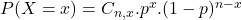In which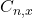is the number of different combinations of x objects from a set of n elements, given by the following formula.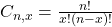And p is the probability of X happening.

​41% of U.S. adults have very little confidence in newspapers.

This means that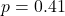You randomly select 10 U.S. adults.

This means that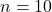(a) exactly​ five

This is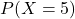. So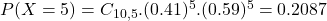0.2087 = 20.82% probability that the number of U.S. adults who have very little confidence in newspapers is exactly​ five.

(b) at least​ six

This is: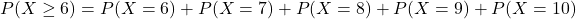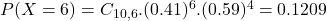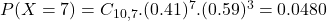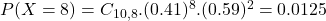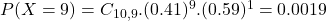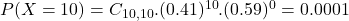Then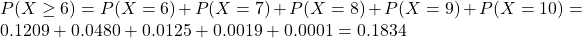0.1834 = 18.34% probability that the number of U.S. adults who have very little confidence in newspapers is at least​ six.

(c) less than four.

This is: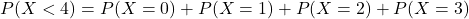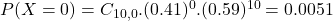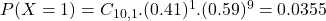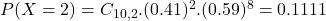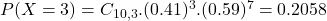So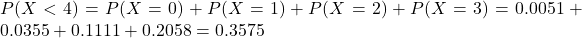0.3575 = 35.75% probability that the number of U.S. adults who have very little confidence in newspapers is less than four.